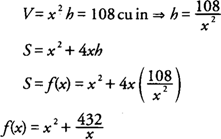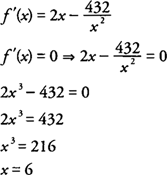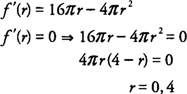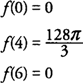## Maximum/Minimum Problems

Many application problems in calculus involve functions for which you want to find maximum or minimum values. The restrictions stated or implied for such functions will determine the domain from which you must work. The function, together with its domain, will suggest which technique is appropriate to use in determining a maximum or minimum value—the Extreme Value Theorem, the First Derivative Test, or the Second Derivative Test.

Example 1: A rectangular box with a square base and no top is to have a volume of 108 cubic inches. Find the dimensions for the box that require the least amount of material.

The function that is to be minimized is the surface area ( S) while the volume ( V) remains fixed at 108 cubic inches (Figure 1) .Figure 1 The open‐topped box for Example 1.

Letting x = length of the square base and h = height of the box, you find thatwith the domain of f(x) = (0,+∞) because x represents a length.hence, a critical point occurs when x = 6. Using the Second Derivative Test:and f has a local minimum at x = 6; hence, the dimensions of the box that require the least amount of material are a length and width of 6 inches and a height of 3 inches.

Example 2: A right circular cylinder is inscribed in a right circular cone so that the center lines of the cylinder and the cone coincide. The cone has 8 cm and radius 6 cm. Find the maximum volume possible for the inscribed cylinder.

The function that is to be maximized is the volume ( V) of a cylinder inscribed in a cone with height 8 cm and radius 6 cm (Figure ) .Figure 2 A cross section of the cone and cylinder for Example 2.

Letting r = radius of the cylinder and h = height of the cylinder and applying similar triangles, you find thatBecause V = π r 2 h and h = 8 −(4/3) r, you find thatwith the domain of f(r) = [0,6] because r represents the radius of the cylinder, which cannot be greater that the radius of the cone.Because f(r) is continuous on [0,6], use the Extreme Value Theorem and evaluate the function at its critical points and its endpoints; hence,hence, the maximum volume is 128π/3 cm 3, which will occur when the radius of the cylinder is 4 cm and its height is 8/3 cm.